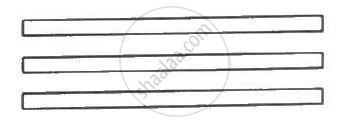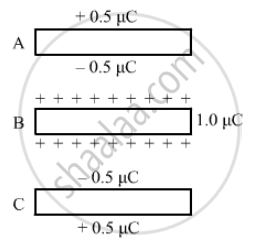Department of Pre-University Education, KarnatakaPUC Karnataka Science Class 12
Share

# The Capacitance Between the Adjacent Plates Shown in Figure is 50 Nf. a Charge of 1⋅0 µC is Placed on the Middle Plate. (A) What Will Be the Charge on the Outer Surface of the Upper Plate? - Physics

ConceptElectric Potential Difference

#### Question

The capacitance between the adjacent plates shown in figure is 50 nF. A charge of 1⋅0 µC is placed on the middle plate. (a) What will be the charge on the outer surface of the upper plate? (b) Find the potential difference developed between the upper and the middle plates.#### Solution(a) When the charge of 1 µC is introduced on plate B, the charge gets equally distributed on its surface. All sides of the plate gets 0.5 µC of charge. Due to induction, 0.5 µC charge is induced on the upper surface of plate A.

(b) Given:-

Capacitance, C = 50 nF = 50 xx 10^-9  "F" = 5 xx 10^-8  "F"

Effective charge on the capacitor, Q_"net" = (1  "uC" - 0)/2 = 0.5  "uC"

∴ Potential difference between plates B and A, V = Q_"net"/C = (5 xx 10^-7)/(5 xx 10^-8) = 10 V

Is there an error in this question or solution?

#### Video TutorialsVIEW ALL 

Solution The Capacitance Between the Adjacent Plates Shown in Figure is 50 Nf. a Charge of 1⋅0 µC is Placed on the Middle Plate. (A) What Will Be the Charge on the Outer Surface of the Upper Plate? Concept: Electric Potential Difference.
S